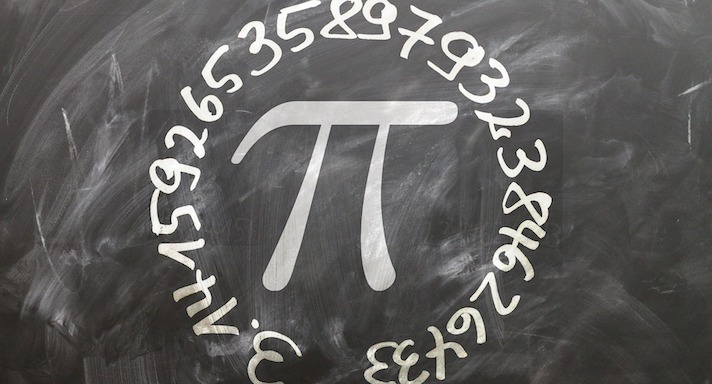# Advanced Algebra I### Course Overview

Acellus Algebra I – Advanced teaches students the basic principles, rules, and operations of working with expressions containing variables.  It is taught by award-winning Acellus Master Teacher, Patrick Mara. Acellus Algebra I - Advanced is A-G Approved through the University of California.

### Course Objectives & Student Learning Outcomes

Upon successful completion of Acellus Algebra I – Advanced, students will have a strong foundation in basic Algebra skills upon which they can build as they delve into more advanced mathematical concepts in future years. Students will know how to solve equations and inequalities and systems of the same. They will be familiar with various types of functions — including linear, quadratic, exponential, and rational functions — and use them to model real world situations. They understand and can identify linear and exponential patterns. Students know what polynomials are and how to factor them. They learn how to model using quadratic equations and have experience working with radical and rational expressions and equations. Students will be familiar with frequency tables, histograms, and box and whisker plots, and will know how to conduct a meaningful survey. They will also know how to calculate permutation and combinations, theoretical and experimental probability, as well as the probability of compound events.This course was developed by the International Academy of Science. Learn More

### Scope and Sequence

Unit 1 – Foundations of Algebra In this unit students discuss variables and expressions, order of operations, real numbers, properties, adding, subtracting, multiplying and dividing, and the distributive property. They are also introduced to equations and two-variable equations. Unit 2 – Equations In this unit students learn about one, two, and multi-step equations, equations with variables on both sides, and literal equations.  They discuss ratios and rates, conversions, similar figures, percents, and percent change. Unit 3 – Inequalities In this unit students explore inequalities, addition, subtraction, multiplication and division with inequalities.  They learn about sets, interval notation, compound inequalities, and absolute value equations and inequalities. Unit 4 – Graphing In this unit students investigate two-variable graphs, patterns, non-linear graphs, graphing a function, and writing a rule.  They explore relations and functions, and arithmetic sequences. Unit 5 – Functions In this unit students study rate of change, direct variation, point-slope, slope-intercept, and standard form.  They investigate parallel and perpendicular, scatter plots and trend line, and absolute value functions. Unit 6 – Systems In this unit students discuss solving systems by graphing, as well as substitution, elimination, and applications of systems.  They also study linear inequalities, and systems of inequalities.  Following this unit students are presented with the Mid-Term Review and Exam. Unit 7 – Exponents In this unit students learn about zero and negative exponents, multiplying powers, multiplication and division properties.  They discuss rational exponents, exponential functions, exponential growth and decay, and geometric sequences. Unit 8 – Polynomials In this unit students explore adding and subtracting polynomials, multiplying and factoring, and multiplying binomials.  They learn about special products, factoring a trinomial, factoring by grouping, and special cases. Unit 9 – Quadratic Functions In this unit students investigate quadratic graphs, functions and equations.  They explore solving by factoring, completing the square, the quadratic formula, math modeling, and systems of linear and quadratic equations. Unit 10 – Radicals In this unit students study the Pythagorean Theorem, simplifying radicals, and operations with radicals.  They investigate solving radical equations, graphing square root functions, and trig ratios. Unit 11 – Rational Expressions In this unit students discuss simplifying, adding, subtracting, multiplying and dividing rational expressions.  They  study dividing polynomials, solving rational equations, inverse variation, and graphing rational functions. Unit 12 – Probability and Statistics In this unit students learn about matrices, frequency and histograms.  They discuss statistical measures, box and whisker plots, samples and surveys, permutations, combinations, theoretical and experimental probability, and compound events.  Following this unit students are presented with the Final Review and Exam.